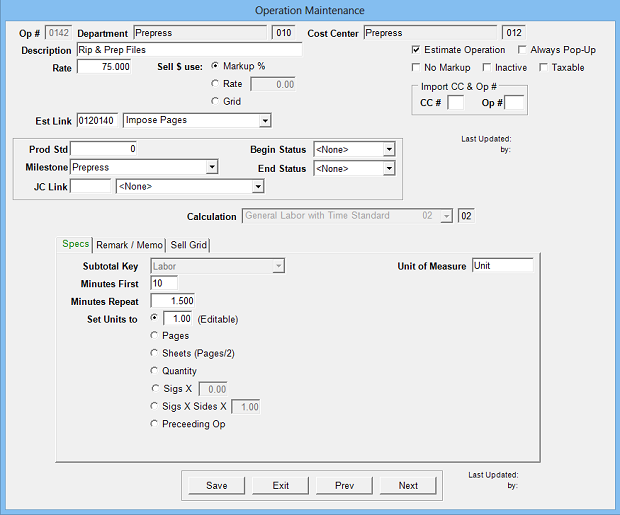Estimates the cost and time for operations where labor standards are used. Minutes per first unit and minutes per repeat unit are time standards.

Operation Setup Requirements:

1. Description
2. Rate: Cost per hour
3. Sell \$ use: Markup % (recommended), alternate sell rate or sell grid
4. Calc: 2
5. Sub Key: Labor
6. Minutes First: Minutes to make first unit
7. Minutes Repeat: Minutes for each additional unit
8. Set units to: Option to default units to pre-assigned number, pages, sheets, form quantity, etc.Estimate Specification Input Prompts:

1. Units (not editable if set to pages, sheets, form quantity, etc.)
2. J%
3. Remarks
4. MemoCalculation:

Minutes First + ((Units - 1) x Minutes Repeat) = Standard Minutes

Standard Minutes / 60 x J% = Estimated Hours

Estimated Hours x Rate = Cost \$

Cost \$ x Markup % = Markup \$

Cost \$ + Markup \$ = Sell \$